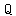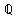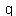# U+211A DOUBLE-STRUCK CAPITAL Q

U+211A was added to Unicode in version 1.1 (1993). It belongs to the blockLetterlike Symbols in the Basic Multilingual Plane.

This character is a Uppercase Letter and is commonly used, that is, in no specific script. The character is also known as the set of rational numbers.

The glyph is a Font composition of the glyphs. It has a Neutral East Asian Width. In bidirectional context it acts as Left To Right and is not mirrored. The glyph can, under circumstances, be confused with 18 other glyphs. In text U+211A behaves as Alphabetic regarding line breaks. It has type Upper for sentence and ALetter for word breaks. The Grapheme Cluster Break is Any.

In mathematics, a rational number is any number that can be expressed as the quotient or fraction p/q of two integers, p and q, with the denominator q not equal to zero. Since q may be equal to 1, every integer is a rational number. The set of all rational numbers is usually denoted by a boldface Q (or blackboard bold , Unicode ℚ); it was thus denoted in 1895 by Peano after quoziente, Italian for "quotient".

The decimal expansion of a rational number always either terminates after a finite number of digits or begins to repeat the same finite sequence of digits over and over. Moreover, any repeating or terminating decimal represents a rational number. These statements hold true not just for base 10, but also for any other integer base (e.g. binary, hexadecimal).

A real number that is not rational is called irrational. Irrational numbers include √2, π, e, and φ. The decimal expansion of an irrational number continues without repeating. Since the set of rational numbers is countable, and the set of real numbers is uncountable, almost all real numbers are irrational.

The rational numbers can be formally defined as the equivalence classes of the quotient set (Z × (Z \ {0})) / ~, where the cartesian product Z × (Z \ {0}) is the set of all ordered pairs (m,n) where m and n are integers, n is not 0 (n ≠ 0), and "~" is the equivalence relation defined by (m1,n1) ~ (m2,n2) if, and only if, m1n2m2n1 = 0.

In abstract algebra, the rational numbers together with certain operations of addition and multiplication form the archetypical field of characteristic zero. As such, it is characterized as having no proper subfield or, alternatively, being the field of fractions for the ring of integers. Finite extensions of Q are called algebraic number fields, and the algebraic closure of Q is the field of algebraic numbers.

In mathematical analysis, the rational numbers form a dense subset of the real numbers. The real numbers can be constructed from the rational numbers by completion, using Cauchy sequences, Dedekind cuts, or infinite decimals.

Zero divided by any other integer equals zero; therefore, zero is a rational number (but division by zero is undefined).

## Representations

System Representation
8474
UTF-8 E2 84 9A
UTF-16 21 1A
UTF-32 00 00 21 1A
URL-Quoted %E2%84%9A
HTML-Escape &#x211A;
Wrong windows-1252 Mojibake â
HTML-Escape &Qopf;
HTML-Escape &rationals;
alias the set of rational numbers
LaTeX \mathbb{Q}

## Complete Record

Property Value
Age (age) 1.1
Unicode Name (na) DOUBLE-STRUCK CAPITAL Q
Unicode 1 Name (na1) DOUBLE-STRUCK Q
Block (blk) Letterlike_Symbols
General Category (gc) Uppercase Letter
Script (sc) Common
Bidirectional Category (bc) Left To Right
Combining Class (ccc) Not Reordered
Decomposition Type (dt) Font
Decomposition Mapping (dm)Lowercase (Lower)
Simple Lowercase Mapping (slc)Lowercase Mapping (lc)Uppercase (Upper)
Simple Uppercase Mapping (suc)Uppercase Mapping (uc)Simple Titlecase Mapping (stc)Titlecase Mapping (tc)Case Folding (cf)ASCII Hex Digit (AHex)
Alphabetic (Alpha)
Bidi Control (Bidi_C)
Bidi Mirrored (Bidi_M)
Bidi Paired Bracket (bpb)Bidi Paired Bracket Type (bpt) None
Cased (Cased)
Composition Exclusion (CE)
Case Ignorable (CI)
Full Composition Exclusion (Comp_Ex)
Changes When Casefolded (CWCF)
Changes When Casemapped (CWCM)
Changes When NFKC Casefolded (CWKCF)
Changes When Lowercased (CWL)
Changes When Titlecased (CWT)
Changes When Uppercased (CWU)
Dash (Dash)
Deprecated (Dep)
Default Ignorable Code Point (DI)
Diacritic (Dia)
East Asian Width (ea) Neutral
Extender (Ext)
FC NFKC Closure (FC_NFKC)Grapheme Cluster Break (GCB) Any
Grapheme Base (Gr_Base)
Grapheme Extend (Gr_Ext)
Hex Digit (Hex)
Hangul Syllable Type (hst) Not Applicable
Hyphen (Hyphen)
ID Continue (IDC)
Ideographic (Ideo)
ID Start (IDS)
IDS Binary Operator (IDSB)
IDS Trinary Operator and (IDST)
InMC (InMC)
Indic Positional Category (InPC) NA
Indic Syllabic Category (InSC) Other
ISO 10646 Comment (isc)
Joining Group (jg) No_Joining_Group
Join Control (Join_C)
Jamo Short Name (JSN)
Joining Type (jt) Non Joining
Line Break (lb) Alphabetic
Logical Order Exception (LOE)
Math (Math)
Noncharacter Code Point (NChar)
NFC Quick Check (NFC_QC) Yes
NFD Quick Check (NFD_QC) Yes
NFKC Casefold (NFKC_CF)NFKC Quick Check (NFKC_QC) No
NFKD Quick Check (NFKD_QC) No
Numeric Type (nt) None
Numeric Value (nv) NaN
Other Alphabetic (OAlpha)
Other Default Ignorable Code Point (ODI)
Other Grapheme Extend (OGr_Ext)
Other ID Continue (OIDC)
Other ID Start (OIDS)
Other Lowercase (OLower)
Other Math (OMath)
Other Uppercase (OUpper)
Pattern Syntax (Pat_Syn)
Pattern White Space (Pat_WS)
Quotation Mark (QMark)
Sentence Break (SB) Upper
Simple Case Folding (scf)Script Extension (scx) Common
Soft Dotted (SD)
STerm (STerm)
Terminal Punctuation (Term)
Unified Ideograph (UIdeo)
Variation Selector (VS)
Word Break (WB) ALetter
White Space (WSpace)
XID Continue (XIDC)
XID Start (XIDS)
Expands On NFC (XO_NFC)
Expands On NFD (XO_NFD)
Expands On NFKC (XO_NFKC)
Expands On NFKD (XO_NFKD)